Impact Factor 3.560 | CiteScore 3.1
More on impact ›

# Frontiers in Physics

## ORIGINAL RESEARCH article

Front. Phys., 20 September 2013 | https://doi.org/10.3389/fphy.2013.00012

# How can we determine if a spacetime is flat?

Valter Moretti* and Roberto Di Criscienzo
• Dipartimento di Matematica, Università di Trento and Istituto Nazionale di Fisica Nucleare - Gruppo Collegato di Trento, Povo, Italy

A spacetime is locally flat if and only if no geodesical deviation exists for congruences of all kinds of geodesics. However, while for causal geodesics the deviation can be measured observing the motion of (infinitesimal) falling bodies, it does not seem possible to evaluate the geodesical deviation of spacelike geodesic. So a physical problem may arise. To tackle this problem we analyze the interplay of local flatness and geodesic deviation measured for causal geodesics. We establish that a generic spacetime is (locally) flat if and only if there is no geodesic deviation for timelike geodesics or, equivalently, there is no geodesic deviation for null geodesics.

## 1. Introduction

The presence of tidal forces, i.e., geodesic deviation for causal geodesics, can be adopted to give a notion of gravitation valid in the general relativistic context, as the geodesic deviation is not affected by the equivalence principle and thus it cannot be canceled out by an appropriate choice of the reference frame. By direct inspection (see 2), one sees that the absence of geodesic deviation referred to all type of geodesics is equivalent the fact that the Riemann tensor vanishes everywhere in a spacetime (M, g). The latter fact, in turn, is equivalent to the locally flatness of the spacetime, i.e., There is a open covering of M,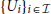, such that every subspacetime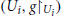is a portion of Minkowski spacetime. However, from a physical viewpoint, the geodesic deviation can easily be measured for causal geodesic, observing the stories of (infinitesimal) falling bodies, but it can hardly be measured for spacelike geodesics. Therefore the popular slogan “gravitation = curvature,” that is “absence of gravitation ⇔ (local) flatness,” seems to encounter an obstruction on the physical ground to be rigorously proved. This is not the case because we establish by Theorem 2.1 that, in a generic spacetime, the absence of geodesic deviation for timelike geodesics – or, equivalently, for null geodesics – is equivalent to the local flatness.

### 1.1. Basic Definitions and Known Results

If ∇ is a C1 affine connection (1; 3) on a C2 manifold M, the curvature tensor (field) is the tensor field defined, point by point, as the unique multi-linear operator Rp : Tp MTp MTp MTp M with:

for every pM and for every triple of C2 vector fields X, Y, Z. It fulfills the following algebraic properties (5), valid for every pM and all Xp, Yp, ZpTpM which will play a role in the rest of the paper.

A result, often mentioned but rarely proved in relativity txtbooks1, establishes that:

Theorem 1.1. Let M be a C2 n-dimensional manifold equipped with a C1 torsionfree affine connection ∇. The curvature tensor vanishes everywhere on M, if and only if M admits an atlas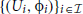such that, the connection coefficients of ∇ vanish in every local chart (Ui, ϕi),

(See the Appendix for a proof). In the following, for a C2 manifold M equipped with a C2 metric g, the symbol ∇ denotes the Levi-Civita connection, i.e., the unique torsionfree, g-metrical (i.e., ∇g = 0), affine connection. The curvature tensor, in this case, is called Riemann (curvature) tensor of g.

## 2. Local Flatness and Geodesic Deviation

A spacetime (M, g) is a smooth (i.e., C) four-dimensional manifold M, equipped with a smooth metric g with Lorentzian signature (−, +, +, +). We state our definition of locally flat spacetime.

Definition 2.1. A spacetime (M, g) is locally flat if it admits a covering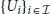made of open subsets, such that every spacetimeis isometric to a spacetime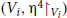, Vi being an open set in Minkowski spacetime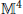and η4 being the standard Minkowski metric of.

The issue about global flatness of a locally flat spacetime (M, g) – i.e., if (M, g) is globally isometric to Minkowski spacetime (, η4) – is of topological nature and will not be addressed here. Locally flat spacetims which are not globally flat can be constructed easily, taking some discrete-isometry-invariant identifications in. From a pure mathematical point of view, Theorem 2.1 immediately implies that:

Lemma 2.1. A spacetime (M, g) is locally flat if and only if the Riemann tensor R vanishes everywhere.

Proof. Locally flatness yields R = 0 everywhere. If R = 0 everywhere, consider the atlas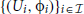of Theorem 1. The components of g are constant in each (Ui, ϕi) since the connection coefficients vanish and ∇g = 0. Using constant linear transformations, one diagonalizes the matrix-valued function representing g in every Ui, obtaining the Minkowskian standard form of the metric in each Ui.

□

As a second step, let us review the notion of geodesic deviation (2; 5) starting with a definition.

Definition 2.2. Consider, in the spacetime (M, g), a pair ({γs}sI, J), such that I, J ⊂ ℝ are open nonempty intervals, for every fixed sI, ${J}{:}{t}{↦}{{\gamma }}_{{s}}{\left(}{t}{\right)}$ is a geodesic, t being a (common) affine parameter, and the map is smooth. Defining ${T}{:}{=}\frac{{\partial }}{{\partial }{t}}$ and ${S}{:}{=}\frac{{\partial }}{{\partial }{s}}$, assume that

Such a pair ({γs}sI, J) will be called a smooth congruence of geodesics.

The constraint (4) assures that, as is physically expected, one can adapt a coordinate system to the smooth class of geodesics, at least locally, such that two coordinates just coincide with t and s. For nonnull geodesics, t can be chosen as the proper length parameter for spacelike geodesics, or the proper time for timelike geodesics. At least when S is spacelike, ∇TS defines the relative speed, referred to the parameter t, between infinitesimally close geodesics (say γs and γs + δs). Similarly, ∇T(∇TS) defines the relative acceleration, referred to the parameter t, between infinitesimally close geodesics. Starting form ∇T(∇TS), employing the definition (1), applying (4), and taking the geodesic equation ∇T T = 0 into account, one finds the geodesic deviation equation:

Let us restrict, from now on, to smooth congruences of causal geodesics with spacelike vectors S, since they have a dynamical interpretation, describing the stories of of free falling bodies, ∇T(∇TS) being the relative acceleration. The presence of tidal forces on free falling bodies, represented by the left-hand side of (5), cannot be canceled by means of a suitable choice of the reference frame, but it is a property of the geometry of the spacetime. Thus, the presence of geodesic deviation for a smooth congruences of causal geodesics with spacelike S can be used to give a sensible, relativistic, definition of gravitation, which is not affected by the equivalence principle. In locally flat spacetimes, where R = 0, there is no geodesic deviation – so that gravitation disappears. It is interesting to study if the absence of geodesic deviation for causal geodesics – i.e., the absence of gravitation – implies the local flatness of the spacetime. It is important to remark that the full information about the curvature may be obtained from the equation of geodesic deviation (5), if considering also smooth congruences of spacelike geodesics (2). However, from the experimentalist's viewpoint, ∇T(∇TS) can hardly be measured along spacelike geodesics, excluding particular cases of spacetimes as static ones, and referring to a very special choice of the field S. For this reason we stick to smooth congruences of causal geodesics with spacelike S only. The following theorem shows that, actually, geodesic deviation of timelike geodesics, or equivalently, geodesic deviation of null geodesics, encodes all information on the curvature.

Theorem 2.1. Consider a spacetime (M, g). The following facts are equivalent.

(a) (M, g) is locally flat;

(b) for every smooth congruence of geodesics ({γs}sI, J) such that, γs is a timelike geodesic and Sγs(t) is spacelike ∀(s, t) ∈ I × J, there is no geodesic deviation, i.e., (∇T(∇T S))γs(t) = 0, for all (s, t) ∈ I × J;

(c) for every smooth congruence of geodesics ({γs}sI, J) such that, γs is a null geodesic and Sγs(t) is spacelike ∀(s, t) ∈ I × J, there is no geodesic deviation, i.e., (∇T(∇TS))γs(t) = 0, for all (s, t) ∈ I × J.

Proof. In view of Lemma 2.1 and of Equation (5), (a) implies both (b) and (c). Let us demonstrate that (b) implies (a). The idea is to prove, making use of (b), (2) and (3), that for each point pM, it holds Rp(Xp, Yp)Zp = 0 for every choice of vectors Xp, Yp, ZpTpM. This is equivalent to say that the Riemann tensor vanishes everywhere on M. At this point, Lemma 2.1 implies (a). Let us proceed step-by-step along this way. Fix pM and assume that (b) is valid. The following lemma holds, whose proof stays in the Appendix.

Lemma 2.2. If (M, g) is a spacetime, pM, let TpTp M\{0} and SpTp M\{0} be, respectively timelike and spacelike, vectors with g(Tp, Sp) = 0. There is a smooth congruence of geodesics ({γs}sI, J) as in (b) of Theorem 2.1, fulfilling Tγs0(t0) = Tp, and Sγs0(t0) = Sp, for some (s0, t0) ∈ I × J.

In view of Lemma 2.2 and Equation (5), one has that, for every pM, Rp(Sp, Tp)Tp = 0 for all Tp, SpTpM, respectively timelike and spacelike, with g(Tp, Sp) = 0. To extend this result to all possible arguments of R, we start noticing that Rp(Sp, Tp)Tp = 0 is still valid if dropping the requirements Sp spacelike and g(Tp, Sp) = 0. Indeed, if SpTpM is generic, we can decompose it as Sp = S'p + cTp, where c ∈ ℝ and S'p is spacelike with g(Tp, S'p) = 0, for some timelike vector Tp. Then Rp(Sp, Tp)Tp = Rp(S'p, Tp)Tp + cRp(Tp, Tp)Tp = 0 + cRp(Tp, Tp)Tp = 0, where we have used Equation (3). Summarizing, (b) implies that Rp(Sp, Tp)Tp = 0 for all Tp, SpTpM with Tp timelike. Let us show that this last constraint can be dropped, too. To this goal, fix SpTpM arbitrarily and consider the bi-linear map . If we restrict FSp to one of the two open halves Vp(+) of the light-cone at p, e.g. that containing the future-directed timelike vectors, we find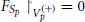in view of the discussion above. Since FSp is analytic (it being a polynomial) and defined on the connected open domain TpM, it must vanish everywhere on TpM. Summarizing, we have obtained that Rp(Sp, Tp)Tp = 0 for every vectors Tp, SpTpM. To conclude, let us prove that the identity above holds true if replacing the latter Tp with a generic vector Zp. Starting from Rp(Sp, Tp)Tp = 0, assuming Tp = Up + Vp and Tp = UpVp, subtracting side-by-side the obtained results, taking bi-linearity into account, one finds:

which is valid for every Sp, Up, VpTpM. Identity (3) can be specialized here as:

Summing side-by-side (6) and (7), taking Equation (2) into account, it arises 2Rp(Sp, Up)Vp + Rp(Up, Vp)Sp = 0, which can be recast as 2Rp(Sp, Up)VpRp(Up, Sp)Vp = 0, where we employed Equation (6) (with different names of the vectors). Using Equation (2) again, we can restate the obtained result as: 2Rp(Sp, Up)Vp + Rp(Sp, Up)Vp = 0. In other words Rp(Sp, Up)Vp = 0 for all vectors Sp, Up, VpTpM, so that Rp = 0 as wanted. This concludes the proof that (b) implies (a), in view of Lemma 2.1.

Let us finally demonstrate that (c) implies (a) by reducing to the proof of the implication (b) ⇒ (a). Fix pM and assume that (c) is valid. The following lemma holds, whose proof stays in the Appendix.

Lemma 2.3. If (M, g) is a spacetime, pM, let TpTp M\{0} and SpTp M\{0} be, respectively timelike and spacelike and with g(Tp, Tp) = − g(Sp, Sp). Defining the null vectors N± := Tp ± Sp, there are smooth congruences of geodesics ({γ±s}sI±, J±) as in (c) of Theorem 2.1, fulfilling Tγ±s0(t0) = N±p, and Sγ±s0(t0) = Sp, for some (s±0, t±0) ∈ I± × J±.

In view of the lemma and of Equation (5), one has that, for every pM, Rp(Sp, N±p)N±p = 0 for all N±p, SpTpM as in the hypotheses of the lemma. Consequently, if Tp and Sp, respectively timelike and spacelike, satisfies g(Tp, Tp) = − g(Sp, Sp), it holds:

where we have used Equation (2), and also

Summing the two expressions found for R(S, T)T we have that, using Equation (2) again:

We have found that Rp(Sp,Tp)Tp = 0 for all Tp, SpTpM, respectively timelike and spacelike, with g(Tp, Sp) = 0 (the requirement g(Tp, Tp) = − gp(Sp, Sp) may be dropped in view of multi-linearity of Rp). Henceforth the proof goes on as in the proof of (b) ⇒ (a) given above.

□

## 3. Summary

We have rigorously analyzed the popular statement “absence of gravitation ⇔ local flatness of the spacetime,” using a notion of gravitation based on the presence of geodesic deviation, measured along congruences of causal geodesics representing the stories of free falling bodies. Spacelike geodesics have not been considered since on a hand they have not dynamical meaning, on the other hand, they are not suitable for direct experimentation in general spacetimes. We have found that, in view of Theorem 2.1 the popular statement holds true. That theorem is interesting also disregarding the definition of relativistic gravitation we have adopted. Indeed it relates, through an if and only if clause, the curvature of a spacetime with purely dynamical properties of free falling bodies which, ideally, may be measured experimentally.

## Conflict of Interest Statement

The authors declare that the research was conducted in the absence of any commercial or financial relationships that could be construed as a potential conflict of interest.

## Footnotes

1 ^A sketch of proof can be found in 2 for Levi-Civita connections.

## References

1. Kobayashi S, Nomizu K. Foundations of Differential Geometry Vol. 1. New York: Interscience Publishers (1963).

2. Minsner CW, Thorne KS, Wheeler JA. Gravitation New York: Freeman (2003).

3. O'Neill B. Semi-Riemannian Geometry with Applications to Relativity New York: Academic Press (1983)

4. Silov GE. MatematiČeskij Analiz Vol.2, Moskow: Nauka (1979).

5. Wald RM. General Relativity Chicago: Chicago University Press (1984).

## Appendix

### Proof of Some Statements

Proof of Theorem2.1. If the connection coefficients vanish in the local chart (Uii), the curvature tensor vanishes therein as well. As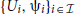is an atlas of M, the curvature tensor vanishes everywhere on M. The converse property is much less trivial to establish. We will exploit the well-known (4):

Frobenius Theorem for first order PDE. Consider the Cauchy problem for the field X: Ω → ℝn,

where F: Ω × ℝm → ℝm, Ω ⊂ ℝn being an open subset, F is a Cs function with s fixed in {1, 2,…} ∪ {∞}, p ∈ Ω, and X0 ∈ ℝm. A unique solution exists in a sufficiently small neighborhood of p, and it is of class Cs + 1 (or C when s = ∞), provided the following conditions are fulfilled in Ω × ℝn

[The conditions (9) are obtained using Schwartz commuting second-order partial derivatives theorem for X and taking (8) into account.] Now consider a C2 manifold M equipped with a C1 torsionfree connection ∇. Fix a point pM and coordinate patch (Ω, ψ) about p, denoting by x ≡ (x1, …, xn) ∈ ℝ the values attained by ψ: Ω → ℝn. From now on, with some obvious misuse of notation, we identify Ω with the corresponding open set in ℝn in order to exploit Frobenius' theorem. We consider the Cauchy problem in Ω × ℝn2 for the composite vector field X ≡ (X(1),…, X(n)), given by

where {Va}a = 1,…, n is a base of TpMTpn ≡ ℝn. Making explicit the covariant derivatives ∇ in the left-hand side in terms of standard coordinate derivatives and connection coefficients (they are C1 functions in our hypotheses), the system of equations takes the form (8) for the composite vector field X. Then, using the expression of the curvature tensor in coordinates, and reminding that the connection is torsionfree, one straightforwardly finds that the condition (9) is valid if the curvature tensor vanishes on Ω. Therefore the vector fields X(a) exist in a neighborhood U' of p. These fields are obtained by parellely transporting the basis of fields V(a) along any curve connecting p and q. Therefore they individuate a basis of TqM at each qU'. Finally, we notice that, in a neighborhood UU' of p, there is a coordinate system ϕ with coordinates y1, …, yn such that $\frac{{\partial }}{{\partial }{{y}}^{{a}}}{=}{{X}}_{{\left(}{a}{\right)}}$ for a = 1, 2,…, n. This is because the distribution of the C2 fields X(1), … X(n) is integrable: as the connection is torsion free, [X(a), X(b)] = ∇X(b) X(a) − ∇X(a) X(b), and ∇X(c) = 0 by hypotheses. The condition ${0}{=}{{\nabla }}_{{{X}}_{{\left(}{b}{\right)}}}{{X}}_{{\left(}{a}{\right)}}{=}{{\nabla }}_{\frac{{\partial }}{{\partial }{{y}}^{{b}}}}\frac{{\partial }}{{\partial }{{y}}^{{a}}}$ says that in the coordinate patch (U, ϕ) the connection coefficients of ∇ vanish. Varying pM, the associated charts (U, ϕ) define the wanted atlas.

□

Proof of Lemma 2.2. Take two spacelike orthogonal vectors Up, VpTpM such that they are also orthogonal with Tp and Sp and consider a normal coordinate system (3) . D ⊂ ℝ4 is a sufficiently small neighborhood of the origin of ℝn. Since $\frac{{\partial }}{{\partial }{{x}}^{{0}}}{{|}}_{{p}}{=}{{T}}_{{p}}$ is timelike, the vectors $\frac{{\partial }}{{\partial }{{x}}^{{0}}}$ have to be timelike by continuity, restricting the domain D sufficiently about the origin. With this restriction, the embedded submanifold Σ through p, individuated by (x0, x1, x2, x3) ∈ D and x0 = 0, turns out to be timelike, x1, x2, x3 are coordinates on Σ and is the (timelike) normal vector at each point of Σ. Finally consider the system of normal coordinates t, s, s2, s3 about Σ (3), individuated by , where q(s, s2, s3) in the right-hand side indicates the point on Σ with coordinates x1 = s, x2 = s2, x3 = s3 and the exponential map is that in M. The open set G is a sufficiently small neighborhood of the origin of ℝ4 which, obviously, can always be taken of the form J × I × I × I, where I, J ⊂ ℝ are open intervals containing the origin of ℝ. The wanted smooth congruence of geodesics is . The vectors ${{S}}_{{{\gamma }}_{{s}}{\left(}{t}{\right)}}{=}\frac{{\partial }}{{\partial }{s}}$ and ${{T}}_{{{\gamma }}_{{s}}{\left(}{t}{\right)}}{=}\frac{{\partial }}{{\partial }{t}}$ are linearly independent and their commutator vanishes because they are tangent to a coordinate system. Tγs(t) is timelike, since it is the tangent vector to geodesics with timelike initial tangent vector . As requested, it also trivially arises that Tγ0(0) = Tp and Sγ0(0) = Sp. As Sp is spacelike, ${{S}}_{{{\gamma }}_{{s}}{\left(}{t}{\right)}}{=}\frac{{\partial }}{{\partial }{s}}$ has to be spacelike everywhere by continuity (shrinking I and J if necessary).

□

Proof of Lemma 2.3. Starting from Tp and Sp, construct the coordinates x0, x1, x2, x3 about the spacelike hypersufrace Σ, individuated by x0 = 0, exactly as in the proof of Lemma 2.2. Next, at each point q ∈ Σ, define the null vectors . Notice that those fields are well-defined and coincides to N±p if q = p. From now on we focus on the N+ case only, the other case being closely similar. Since N+ is nowhere tangent to Σ by construction, one may define a system of null-Riemannian coordinates t, s, s2, s3 about Σ (3), individuated by the expression , where q(s, s2, s3) in the right-hand side indicates the point on Σ with coordinates x1 = s, x2 = s2, x3 = s3 and the exponential map is that in M. The open set G is a sufficiently small neighborhood of the origin of ℝ4 which, obviously, can always be taken of the form J+ × I+ × I+ × I+, where I+, J+ ⊂ ℝ are open intervals containing the origin of ℝ. The wanted smooth congruence of geodesics is γ+s(t) := expq(s, 0, 0) (t N+q(s, 0, 0)). The vectors ${{S}}_{{{\gamma }}_{{s}}^{{+}}{\left(}{t}{\right)}}{=}\frac{{\partial }}{{\partial }{s}}$ and ${{T}}_{{{\gamma }}_{{s}}^{{+}}{\left(}{t}{\right)}}{=}\frac{{\partial }}{{\partial }{t}}$ are linearly independent and their commutator vanishes because they are tangent to a coordinate system. Tγ+s(t) is null, since it is the tangent vector to geodesics with null initial tangent vector N+(0, s, 0, 0). As requested, it also trivially arises that Tγ+0(0) = N+p and Sγ+0(0) = Sp. As Sp is spacelike, by continuity (shrinking I+ and J+ if necessary), ${{S}}_{{{\gamma }}_{{s}}{\left(}{t}{\right)}}{=}\frac{{\partial }}{{\partial }{s}}$ has to be spacelike everywhere it is defined.

□

Keywords: general relativity, geodesical deviation, local flatness, causal geodesic, riemann tensor

Citation: Moretti V and Di Criscienzo R (2013) How can we determine if a spacetime is flat? Front. Physics 1:12. doi: 10.3389/fphy.2013.00012

Received: 10 August 2013; Accepted: 31 August 2013;
Published online: 20 September 2013.

Edited by:

Mauro Spreaficom, University of São Paulo, Brazil

Reviewed by:

Yutaka Shikano, Institute for Molecular Science, Japan
Igor Mencattini, University of São Paulo, Brazil

Copyright © 2013 Moretti and Di Criscienzo. This is an open-access article distributed under the terms of the Creative Commons Attribution License (CC BY). The use, distribution or reproduction in other forums is permitted, provided the original author(s) or licensor are credited and that the original publication in this journal is cited, in accordance with accepted academic practice. No use, distribution or reproduction is permitted which does not comply with these terms.

*Correspondence: Valter Moretti, Dipartimento di Matematica, Università di Trento and Istituto Nazionale di Fisica Nucleare - Gruppo Collegato di Trento, via Sommarive 14 I-38050 Povo, Italy e-mail: moretti@science.unitn.it